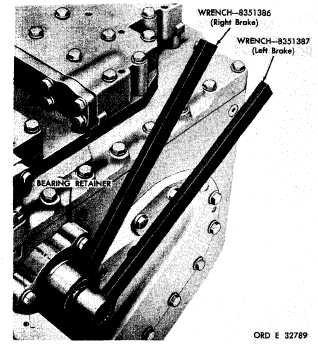Custom SearchP A R    2 3 3 - 2 3 4 T  E  S  T  S      A  N  D      A  D  J  U  S  T  M  E  N  T S C H A P   5,    SEC    X X X I V brakes   will   stop   such   rotation   when   the   power train   is   functioning   properly. (2)   In   all   four   forward   gears,   when   no steer   is   applied,   the   power   train   outputs   should r o t a t e    c l o c k w i s e ,    a s    v i e w e d    f r o m    t h e    r i g ht side   of   the   power   train. (3)   In   both   reverse   gears,   when   no   steer is   applied,   the   power   train   outputs   should   ro- t a t e    c o u n t e r c l o c k w i s e ,    a s    v i e w e d    f r o m    t h e right   side   of   the   power   train. (4)   In   forward   gears   (first   and   second), i n    c l u t c h - b r a k e    f u l l - r i g h t    s t e e r ,    t h e    r i g h t power   train   output   should   stop   while   the   left- output   rotates   clockwise,   as   viewed   from   the right   side   of   the   power   train. (5)   In   forward   gears   (first   and   second), in   clutch-brake   full-left   steer,   the   left-power train   output   should   stop   while   the   right   output r o t a t e s    c l o c k w i s e ,    a s    v i e w e d    f r o m    t h e    r i g h t side   of   the   power   train. (6)   In   forward   gears   (third   and   fourth), i n    g e a r e d    f u l l - r i g h t    s t e e r ,    b o t h    p o w e r    t r a in o u t p u t s     s h o u l d     r o t a t e     c l o c k w i s e ,     a s     v i e w ed Figure  370.  Right-  and  left-brake  adjusting  wrenches, properly   positioned f r o m    t h e    r i g h t    s i d e    o f    t h e    p o w e r    t r a i n .    T he l e f t    o u t p u t    s h o u l d    r o t a t e    0 . 4 7 7    t i m e s    f a s t er than   the   right. (7)   In   forward   gears   (third   and   fourth), in   geared   full-left   steer,   both   power   train   out- puts   should   rotate   clockwise,   as   viewed   from the   right   side   of   the   power   train.   The   right output   should   rotate   0.477   times   faster   than the   left. ( 8 )     I n     r e v e r s e     1 ,  clutch-brake    full- steer,   the   right   output   should   stop   when   steer- i n g    r i g h t .    T h e    l e f t    o u t p u t    s h o u l d    s t o p    w h en steering   left.   The   opposite   output   should   ro- t a t e    c o u n t e r c l o c k w i s e ,    a s    v i e w e d    f r o m    t he right   side   of   the   power   train. (9)   In   reverse   2,   geared   full-steer,   the right   output   should   slow   during   right   steer. The   left   output   should   slow   during   left   steer. R o t a t i o n    o f    b o t h    o u t p u t s    s h o u l d    b e    c o u n t e r- c l o c k w i s e ,    a s    v i e w e d    f r o m    t h e    r i g h t    s i d e    of the   power   train. 2 3 4 .     A D J U S T M E N T S .   B r a k e    L i n k a g e (1)   Disconnect   the   vehicle   brake   linkage from   the   transmission.   Check   vehicle   linkage to   see   that   it   is   not   binding. ( 2 )    P o s i t i o n    t h e    v e h i c l e    b r a k e    c o n t r o l in   fully   released   position.   Adjust   vehicle   link- age   until   it   can   be   freely   connected   to   the   trans- m i s s i o n . Secure   the   linkage. .   B r a k e s (1)   Power   train   installed   in   vehicle   and i n    s e r v i c e. )   B r a k e    l i n k a g e    m u s t    b e    p r o p e r ly a d j u s t e d    a s    o u t l i n e d    i n    ,   a b o v e ,    b e f o r e    a t- tempting   this   brake   adjustment   when   the   brake apply   levers   (components   of   brake   linkage)   are c o n n e c t e d . b)    If   brake   apply   levers   are   connect- e d ,    p e r m a n e n t l y    s c r i b e    o r    t e m p o r a r i l y    m a rk both   levers   so   that   the   marks   aline   with   the Release   mark   on   the   bearing   retainer   (fig.   370). 1 9 9Integrated Publishing, Inc. - A (SDVOSB) Service Disabled Veteran Owned Small Business